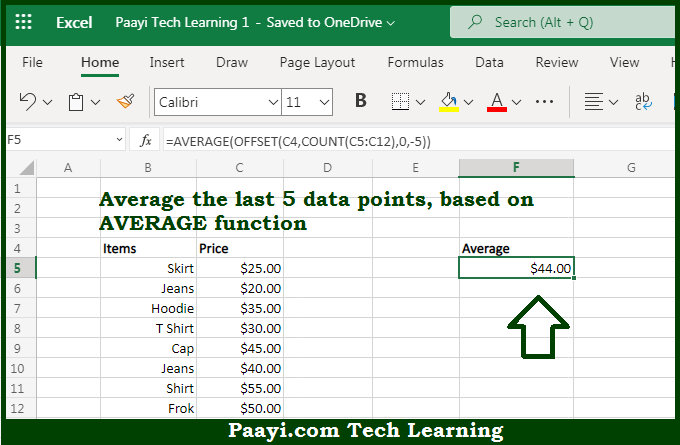# Learn How to AVERAGE Last 5 Values in Microsoft Excel

Written by | 0 Comments | 629 Views

In this article, you will learn how to SUM various things in Microsoft Excel using a single/combination(s) of functions. You will also know how to AVERAGE the Last 5 Values and see the generic formula.

AVERAGE Last 5 Values in Microsoft Excel

The main purpose of this formula is to average the last 5 data points. Here we will learn how to average the last 5 values in the workbook in Microsoft Excel. That implies, with the help of a formula based on the AVERAGE, COUNT, and OFFSET function you can able to average the last 5 data points. So, with the help of this formula, you can able to average the last 5 values in the workbook in Microsoft Excel.

General Formula to AVERAGE Last 5 Values

=AVERAGE(OFFSET(A1,COUNT(A:A),0,-N))

The Explanation for the AVERAGE Last 5 ValuesSo we know that with the help of the given formula above you can able to average the last 5 data points. Here we will learn how to average the last 5 values in the workbook in Microsoft Excel. As we know that the OFFSET function is utilized to construct dynamic rectangular ranges based on a starting reference and given rows, columns, height, and width. Besides that the rows and columns arguments function like "offsets" from the starting reference. It should be noted that the height and width arguments and both are optional and used to determine how many rows and columns the final range includes. So, with the help of this formula, you can able to average the last 5 values in the workbook in Microsoft Excel.